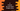# C program to reverse a user input integer array### C program to reverse a user input integer array :

In this tutorial, we will learn how to reverse all values of an integer array using C programming language. For example, the array [1,2,3,4,5] will become [5,4,3,2,1] after reversed. The array elements will be entered by the user. We will store them in an array and reverse the array. The algorithm that is going to be used in the program is as below :

### Algorithm :

1. Ask the user how many numbers the array will contain.
2. Create one array and get values for the array from the user.
3. Start swapping the first and the last elements of the array. For example, for [1,2,3,4,5,6,7] first swap 1 and 7,next swap 2 and 6, next 3 and 5 etc. __4. Do this till no swapping left. This should be the reversed array.
4. Print out the result.__ __

Using the above algorithm, for the example [1,2,3,4,5,6,7],the steps will be as below :

``````Start : [1,2,3,4,5,6,7]
First swap : [7,2,3,4,5,6,1]
Second swap : [7,6,3,4,5,2,1]
Third swap : [7,6,5,4,3,2,1] which is the result.
``````

### C program to reverse an integer array :

``````#include <stdio.h>

int main()
{
//1
int total;
int i;
//2
int start, end, temp;

//3
printf("How many numbers you want to enter : ");
scanf("%d", &total);

//4
int array[total];

//5
for (i = 0; i < total; i++)
{
printf("Enter element for position %d : ", i + 1);
scanf("%d", &array[i]);
}

//6
printf("Array entered : \n");
for (i = 0; i < total; i++)
{
printf("%d ", array[i]);
}

//7
start = 0;
end = total - 1;

//8
while (start < end)
{
temp = array[start];
array[start] = array[end];
array[end] = temp;
start++;
end--;
}

//9
printf("\nArray after reversing is done : \n");
for (i = 0; i < total; i++)
{
printf("%d ", array[i]);
}
}``````

### Explanation :

The commented numbers in the above program denote the step-number below :

1. Create one integer total to store the total numbers for the array . Integer i will be use in loop.
2. start and end will point to the current start and end position of the array . temp to hold value temprarily while swapping.
3. Ask the user how many numbers he wants to enter . Read it and save it in total.
4. Create one integer array with same size as total.
5. Run one for loop to read each elements for the array. Read and save it in the array.
6. Print out the array entered by the user.
7. Set the value of start as 0 i.e. the first element position of the array and end as total - 1 i.e. the index of the last element of the array.
8. Run one while loop and start swapping first and the last element of the array. The loop will run till start < end i.e. till all swapping is completed.Increment the value of start and decrement the value of end after current swapping is done.
9. Print out the result after the loop is completed.

### Sample Output :

``````How many numbers you want to enter : 5
Enter element for position 1 : 1
Enter element for position 2 : 2
Enter element for position 3 : 3
Enter element for position 4 : 4
Enter element for position 5 : 5
Array entered :
1 2 3 4 5
Array after reversing is done :
5 4 3 2 1

How many numbers you want to enter : 7
Enter element for position 1 : 7
Enter element for position 2 : 3
Enter element for position 3 : 5
Enter element for position 4 : 1
Enter element for position 5 : 0
Enter element for position 6 : 4
Enter element for position 7 : 2
Array entered :
7 3 5 1 0 4 2
Array after reversing is done :
2 4 0 1 5 3 7
``````